Next: Vector Surface Integrals Up: Vectors and Vector Fields Previous: Vector Line Integrals

# Surface Integrals

Let us take a surface, that is not necessarily co-planar, and divide it up into (scalar) elements. Then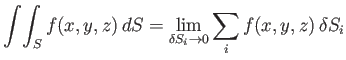(A.84)

is a surface integral. For instance, the volume of water in a lake of depth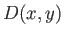is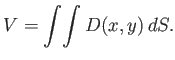(A.85)

To evaluate this integral, we must split the calculation into two ordinary integrals. The volume in the strip shown in Figure A.17 is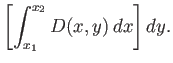(A.86)

Note that the limitsand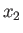depend on. The total volume is the sum over all strips: that is,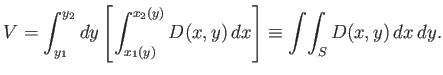(A.87)

Of course, the integral can be evaluated by taking the strips the other way around: that is,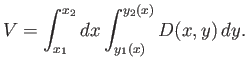(A.88)

Interchanging the order of integration is a very powerful and useful trick. But great care must be taken when evaluating the limits.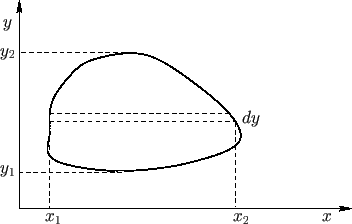For example, consider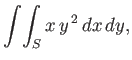(A.89)

whereis shown in Figure A.18. Suppose that we evaluate theintegral first:(A.90)

Let us now evaluate theintegral: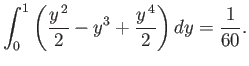(A.91)

We can also evaluate the integral by interchanging the order of integration: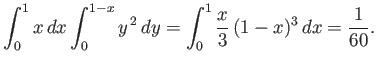(A.92)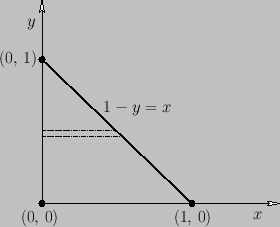In some cases, a surface integral is just the product of two separate integrals. For instance,(A.93)

whereis a unit square. This integral can be written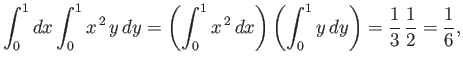(A.94)

because the limits are both independent of the other variable.Next: Vector Surface Integrals Up: Vectors and Vector Fields Previous: Vector Line Integrals
Richard Fitzpatrick 2016-03-31